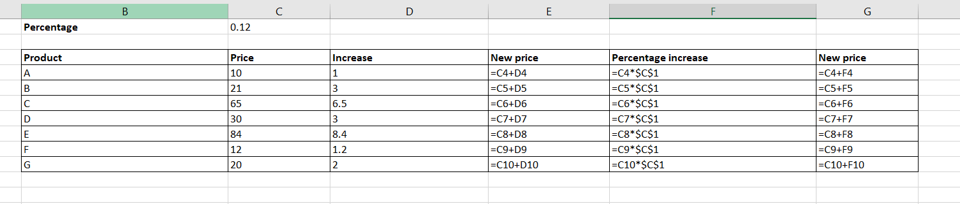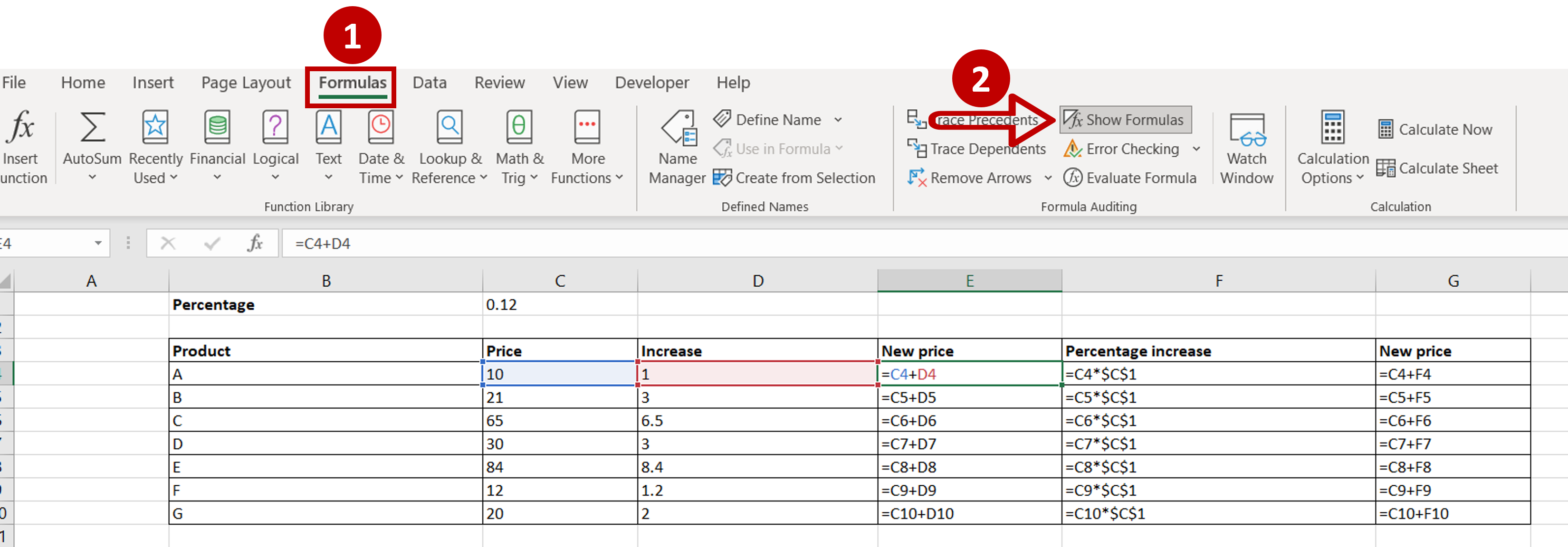# How to show formulas in Excel

You can watch a video tutorial here.Excel is widely used for calculations and can be used to create complex formulas. When a formula is created in a cell, the result of the formula is visible in the cell. To see the formula, you need to select the cell and check the formula bar. When studying an Excel sheet with a lot of columns that have calculated values, you may want to see the formulas in the sheet itself to understand how the calculations have been done.

### Step 1 – Check if the cells have formulas– Select a cell
– Check the Formula bar to see if it contains a formula

### Step 2 – Show the formulas– On the Formulas menu, choose the Show Formulas option